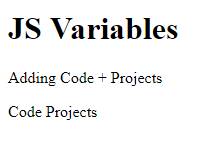# Variables – JavaScript Basics

Variables are the packages for storing the values. Lets take an example, suppose a,b,c are variables :

```<!DOCTYPE html>
<html>
<body>

<h1>JS Variables</h1>
<p> (a, b & c are variables!!) </p>
<p id="ex"></p>

<script>
var a = 10;
var b = 20;
var c = a + b;
document.getElementById("ex").innerHTML =
"Value Of C is = " + c;
</script>

</body>
</html>```

Output :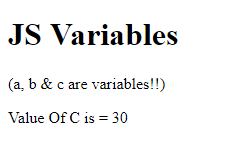JS Identifiers

With unique names all the JS variables should be identified. And these Unique names are known as Identifiers, it can be short name (like a and b) or more descriptive names (age, sum, totalVolume).

1. Reserved words can’t be used as names.
2. Names can contain digits, letters, dollar signs, and underscore.
3. Names must begin with a letter
4. Names are case sensitive (a & A are different variables)
5. Names can also begin with( \$ ) and ( _ )

Data Types

JS handles many types of data, for now lets just think of strings & numbers. Inside double or single quotation (” ” or ‘ ‘ ) Strings are written and numbers are written without quotation. If numbers are kept in a quotation mark then it will be read as a Text!!

```<!DOCTYPE html>
<html>
<body>

<h1>JS Variables</h1>
<p>Strings are written inside Quotation sign & Numbers are written without it!</p>
<p id="ex"></p>

<script>
var pi = 2.22;
var person = "Code Projects";
var answer = 'Projects, Tutorials & More. . ';

document.getElementById("ex").innerHTML =
pi + "<br>" + person + "<br>" + answer;
</script>

</body>
</html>```

Output :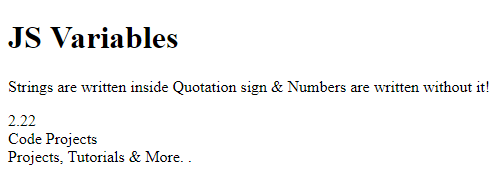Creating Variables

You can simply create a variable with var keyword.

`var Name;`

It has no value; its undefined. ( = ) sign is used to allow value to variable.

```var Name = "Johnny";

Name = "Johnny";```

Now Let’s take an example :

```<!DOCTYPE html>
<html>
<body>

<h1>JS Variables</h1>
<p>Create a variable and then put the value!</p>
<p id="ex"></p>

<script>
var Name = "Johnny";
document.getElementById("ex").innerHTML = Name;
</script>

</body>
</html>```

Output :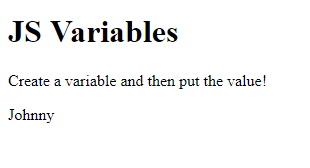Many Variables in One Statement

You can simply create many variables in one statement.

```<!DOCTYPE html>
<html>
<body>

<h1>JS Variables</h1>
<p>Create many variables in one statement!</p>
<p id="ex"></p>

<script>
var person = "Johnny", bikeName = "Yamaha", price = 300000;
document.getElementById("ex").innerHTML = bikeName;
</script>

</body>
</html>```

Output :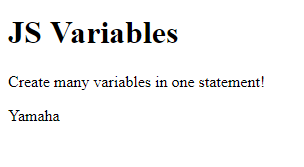Undefined Value

Variable created without a value will have undefined valueLets take an example of variable bikeName which will have undefined value!

```<!DOCTYPE html>
<html>
<body>

<h1>JS Variables</h1>
<p>Variables created without value will have undefined value!</p>
<p id="ex"></p>

<script>
var bikeName;
document.getElementById("ex").innerHTML = bikeName;
</script>

</body>
</html>```

Output :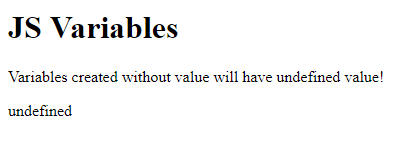Re-Creating Variables

You will not lose its value if you are re-creating same variable!

```<!DOCTYPE html>
<html>
<body>

<h1>JS Variables</h1>
<p>Values wont erase if you are recreating same variable</p>
<p id="ex"></p>

<script>
var bikeName = "Yamaha";
var bikeName;
document.getElementById("ex").innerHTML = bikeName;
</script>

</body>
</html>```

Output :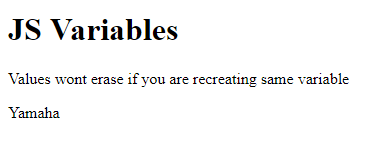Arithmetic

Using operators like ( = ) and ( + ) you can perform arithmetic operations here:

```<!DOCTYPE html>
<html>
<body>

<h1>JS Variables</h1>
<p>Adding 50 + 12 + 22 </p>
<p id="ex"></p>

<script>
var a = 50 + 12 + 22;
document.getElementById("ex").innerHTML = a;
</script>

</body>
</html>```

Output :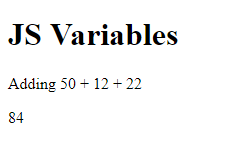```<!DOCTYPE html>
<html>
<body>

<h1>JS Variables</h1>
<p id="ex"></p>

<script>
var a = "Code" + " " + "Projects";
document.getElementById("ex").innerHTML = a;
</script>

</body>
</html>```

Output :